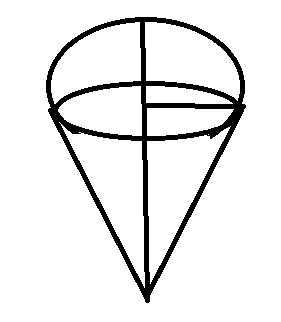Courses
Courses for Kids
Free study material
Free LIVE classes
MoreLIVE
Join Vedantu’s FREE Mastercalss

# Right circular cylinder having diameter $12cm$ and height $15cm$ is full ice-cream. The ice-cream is to be filled in cones of height $12cm$ and diameter $6cm$ having a hemispherical shape on top. Find the number of such cones which can be filled with ice-cream.Verified
363.3k+ views
Hint: To find the number of cones, first we will find the volume of the circular cylinder and divide it with the volume of one ice-cream cone.

So,
Number of cones volume of the circular cylinder ÷ volume of one ice-cream cone
= $\dfrac{{\pi \times 6 \times 6 \times 15}}{{\dfrac{1}{3} \times \pi \times 3 \times 3 \times 12 + \dfrac{{2\pi }}{3} \times {3^2}}} = \dfrac{{\pi \times 36 \times 15}}{{\dfrac{\pi }{3} \times \left( {108 + 54} \right)}} = 10$
Answer $10$ cones

Note: In these questions, make sure you take the volume of the entities and not the area or perimeter.
Remembering the volume formulas is often helpful.
Last updated date: 23rd Sep 2023
Total views: 363.3k
Views today: 4.63k